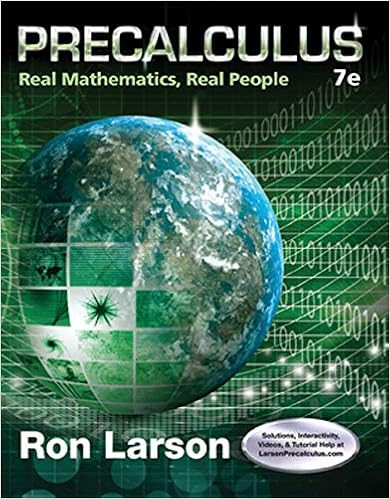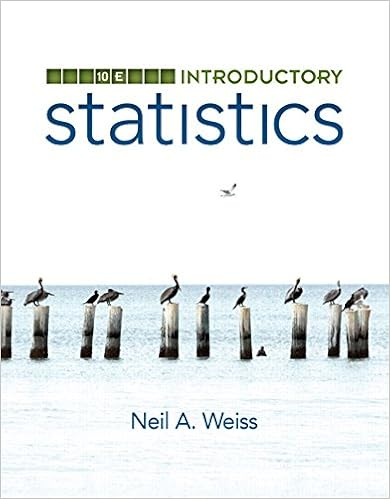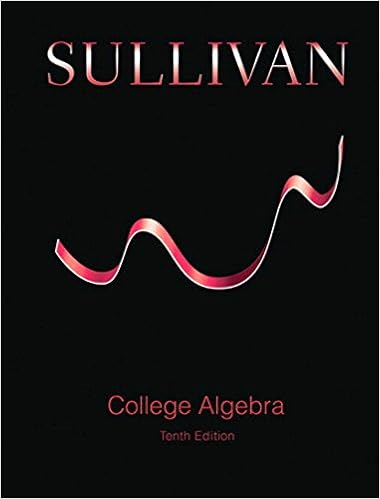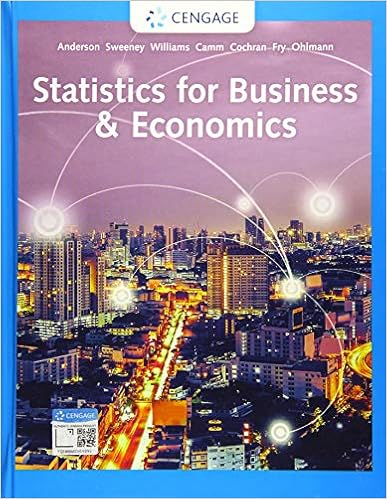# MCV4U_Unit#6_TCavers.docx - MCV4U: Lesson #16-17 Lines and...

• 16
• 100% (10) 10 out of 10 people found this document helpful

This preview shows page 1 - 6 out of 16 pages.

The preview shows page 4 - 6 out of 16 pages.
MCV4U: Lesson #16-17 –Lines and Planes (Part A)Taylor CaversStudent Number: 17144011
##### We have textbook solutions for you!The document you are viewing contains questions related to this textbook.
Chapter 7 / Exercise 2
Precalculus: Real Mathematics, Real People
LarsonExpert VerifiedBrowse all Textbook Solutions
Lesson #17: Application of Vector MultiplicationTask #1: Knowledge and Understanding Questions1.Find the angle between vectorsa=(7,1,2)andb=(5,4,4). (5 marks)aab
cosθ=313078cosθ=0.5588θ=cos1(0.5588)θ=56.0°Therefore, the angle between vectorsa=(7,1,2)andb=(5,4,4)is 56.0° and isacute.2.Find the cross product ofu=(−2,3,4)andv=(1,4,5). (4 marks)is
3.Given two vectorsu=(5,1,5)andv=(−4,5,2), find the projection ofuvin Cartesian form. (4 marks)on
Projvu=(43,53,23)Therefore, the projection ofuonvin Cartesian form isProjvu=(43,53,23).4.Find the volume of a parallelepiped defined by the vectorsu=(3,4,2)v=(0,2,3), andw=(−2,0,4). (4 marks),.
Therefore, the volume of a parallelepiped defined by the vectorsu=(3,4,2),v=(0,2,3), andw=(−2,0,4)isV=56cubic units.

Course Hero member to access this document

Course Hero member to access this document

End of preview. Want to read all 16 pages?

Course Hero member to access this document

Term
Summer
Professor
N/A
Tags
##### We have textbook solutions for you!
The document you are viewing contains questions related to this textbook.The document you are viewing contains questions related to this textbook.
Chapter 7 / Exercise 2
Precalculus: Real Mathematics, Real People
LarsonExpert Verified
•••